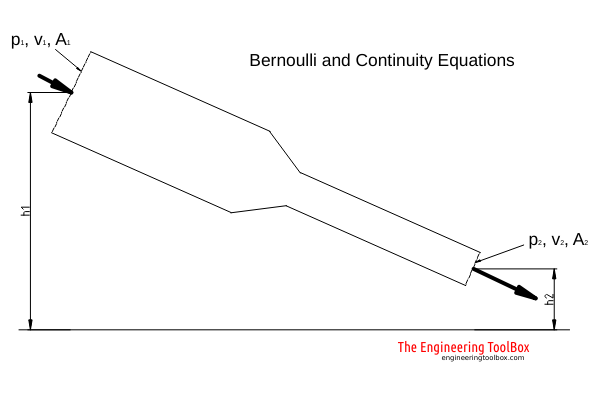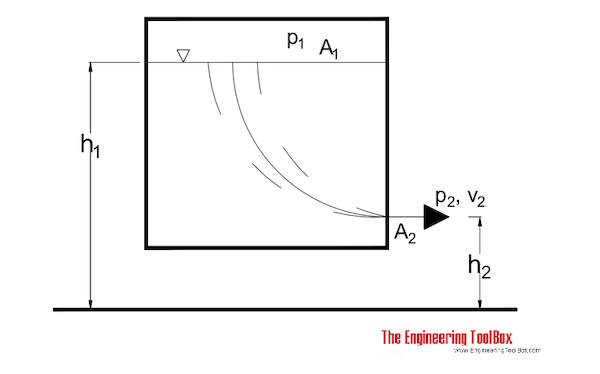Engineering ToolBox - Resources, Tools and Basic Information for Engineering and Design of Technical Applications!

# Bernoulli Equation

## Conservation of energy in a non-viscous, incompressible fluid at steady flow.

The statement of conservation of energy is useful when solving problems involving fluids. For a non-viscous, in-compressible fluid in a steady flow, the sum of pressure, potential and kinetic energies per unit volume is constant at any point.

Bernoulli's principle: At points along a horizontal streamline, higher pressure regions have lower fluid speed and lower pressure regions have higher fluid speed.

A special form of the Euler’s equation derived along a fluid flow streamline is often called the Bernoulli Equation:### Energy Form

For steady state in-compressible flow the Euler equation becomes

E = p1 / ρ + v12 / 2 + g h1

= p2 / ρ + v22 / 2 + g h2 - Eloss

= constant                    (1)

where

E = energy per unit mass in flow (J/kg, Btu/slug)

p = pressure in the fluid (Pa, psf)

ρ = density of fluid (kg/m3, slug/ft3)

v = velocity of fluid (m/s, ft/s)

Eloss = energy loss per unit mass in flow (J/kg, Btu/slug)

1 psi = 144 psf

(1) can be modified by dividing with gravity like

h = p1 / γ + v12 / (2 g) + h1 = p2γ + v22 / (2 g) + h2 - Eloss / g = constant                     (2)

where

h = head (m fluid column, ft fluid column)

γ = ρ g = specific weight of fluid (N/kg, lbf/slug)

Equation (2) is often referred to as the "head" because all elements has the unit of length.

Note! - the head unit is with reference to the density of the flowing fluid. For other units - like mm Water Column - check Velocity Pressure Head.

### Dynamic Pressure

(1) and (2) are two forms of the Bernoulli Equation for a steady state in-compressible flow. If we assume that the gravitational body force is negligible - the elevation is small - then the Bernoulli equation can be modified to

p = p1 + ρ v12 / 2

= p2 + ρ v22 / 2 - ploss

= p1 + pd1 = p2 + pd2 - ploss                             (3)

where

p = pressure (Pa, psi)

ploss = pressure loss (Pa, psi)

pd = 1/2 ρ v= dynamic pressure (Pa, psf)

It is common to refer to the flow velocity component as the dynamic pressure of the fluid flow.

Note! - increased flow velocity reduces pressure - decreased flow velocity increases pressure.

This phenomena can be observed in a venturi meter where the pressure is reduced in the constriction area and regained after. It can also be observed in a pitot tube where the stagnation pressure is measured. The stagnation pressure is where the velocity component is zero.

### Bernoulli Equation and Flow from a Tank through a small Orifice

Liquid flows from a tank through a orifice close to the bottom. The Bernoulli equation can be adapted to a streamline from the surface (1) to the orifice (2):p1 / γ + v12 / (2 g) + h1

= p2γ + v22 / (2 g) + h2 - Eloss / g              (4)

By multiplying with g and assuming that the energy loss is neglect-able - (4) can be transformed to

p1 / ρ + v12 / 2  + g h1

= p2ρ + v22 / 2  + g h2              (4b)

#### Discharge Velocity

If

h = h1 - h2                     (4c)

and (according the continuity equation)

v1 = (A2 / A1) v2                     (4d)

then the velocity out of the orifice can be expressed as

v2 = ([2 / (1 - A22 / A12)][g h + (p1 - p2) / 2])1/2                 (5)

#### Vented Tank

For a vented tank where the inside pressure equals the outside pressure

p1 = p2                              (5b)

and the surface area is much larger than the orifice area

A1 >> A2                     (5c)

- then eq. 5 can be modified to

v2 = (2 g h)1/2                      (6)

"The velocity out from the tank is equal to speed of a freely body falling the distance h." - also known as Torricelli's Theorem.

##### Example - outlet velocity from a vented tank

The outlet velocity from a tank with level 10 m can be calculated as

v2 = (2 (9.81 m/s2) (10 m))1/2

= 14 m/s

#### Orifice Discharge Coefficient

Eq. 6 is for ideal flow without pressure loss in the orifice. In the real worls - with pressure loss - eq. 6 can be expressed with a coefficient of discharge - friction coefficient - as

v2 = c (2 g h)1/2                      (6b)

where

c = coefficient of discharge

The coefficient of discharge can be determined experimentally. For a sharp edged opening it may bee as low as 0.6. For smooth orifices it may bee between 0.95 and 1.

#### Pressurized Tank

If the tank is closed, pressurized and the level between the surface and the discharge outlet minimal (the influence from level difference is very small compared to pressure influence in eq. 5) - the discharge velocity can be expressed as

v2 = c (2 (p1 - p2) / ρ)1/2                       (7)

##### Example - Outlet Velocity from a Pressurized Tank

The outlet velocity of a pressurized tank where

p1 = 0.2 (MN/m2, 106 Pa)

p2 = 0.1 (MN/m2, 106 Pa)

A2 / A1 = 0.01

h = 10 (m)

can be calculated as

V2 = ( (2 / (1 - (0.01)2) ((0.2 106 N/m2) - (0.1 106 N/m2)) / (1000 kg/m3) + (9.81 m/s2) (10 m)))1/2

= 19.9 m/s

### Energy Loss through a Reduction Valve

When fluid flows through a reduction valve and the pressure is reduced - there is an energy loss. By neglecting the change in elevation (h1 = h2) and the change in fluid velocity (v1 = v2) the pressure energy before the valve and the pressure energy after the valve including the energy loss through the valve - is constant. The Bernouilli equation can be modified to

p1 / ρ = p2 / ρ + Eloss                                       (8)

where

Eloss  = energy loss through valve (J)

(8) can be transformed to:

Eloss  = (p1 - p2) / ρ                        (8b)

## Related Topics

• ### Flow Measurement

Flow metering principles - Orifice, Venturi, Flow Nozzles, Pitot Tubes, Target, Variable Area, Positive Displacement, Turbine, Vortex, Electromagnetic, Ultrasonic Doppler, Ultrasonic Time-of-travel, Mass Coriolis, Mass Thermal, Weir V-notch, Flume Parshall and Sluice Gate flow meters and more.
• ### Fluid Mechanics

The study of fluids - liquids and gases. Involving velocity, pressure, density and temperature as functions of space and time.

## Related Documents

• ### Energy

Energy is the capacity to do work.
• ### Energy and Hydraulic Grade Line

The hydraulic grade line and the energy line are graphical presentations of the Bernoulli equation.
• ### Equations in Fluid Mechanics

Equations used in fluid mechanics - like Bernoulli, conservation of energy, conservation of mass, pressure, Navier-Stokes, ideal gas law, Euler equations, Laplace equations, Darcy-Weisbach Equation and more.
• ### Fluid Flow - Equation of Continuity

The Equation of Continuity is a statement of mass conservation.
• ### Fluid Flowmeters - Comparing Types

An introduction to the different types of fluid flowmeters - Orifices, Venturies, Nozzles, Rotameters, Pitot Tubes, Calorimetrics, Turbine, Vortex, Electromagnetic, Doppler, Ultrasonic, Thermal, Coriolis.
• ### Liquid Flow from Containers - Emptying Time

Calculate liquid velocity, volume flow and draining time when emptying a container.
• ### Mechanical Energy Equation vs. Bernoulli Equation

The Mechanical Energy Equation compared to the Extended Bernoulli Equation.
• ### Pitot Tubes

Pitot tubes can be used to measure fluid flow velocities by measuring the difference between static and dynamic pressure in the flow.
• ### Sluice Gate - Volume Flow Measurements

Sluice gates can be used to control and measure volume flow rates in open channels and rivers, mainly in connection to hydro power plants.
• ### Steam Flow - Orifices

Steam flow through orifices - for steam pressures ranging 2 - 300 psi
• ### Steam Leaks through Orifices

Steam leaks through orifices for pressures ranging 250 - 600 psi

## Engineering ToolBox - SketchUp Extension - Online 3D modeling!

Add standard and customized parametric components - like flange beams, lumbers, piping, stairs and more - to your Sketchup model with the Engineering ToolBox - SketchUp Extension - enabled for use with older versions of the amazing SketchUp Make and the newer "up to date" SketchUp Pro . Add the Engineering ToolBox extension to your SketchUp Make/Pro from the Extension Warehouse !

We don't collect information from our users. More about

## Citation

• The Engineering ToolBox (2003). Bernoulli Equation. [online] Available at: https://www.engineeringtoolbox.com/bernouilli-equation-d_183.html [Accessed Day Month Year].

Modify the access date according your visit.

9.29.12